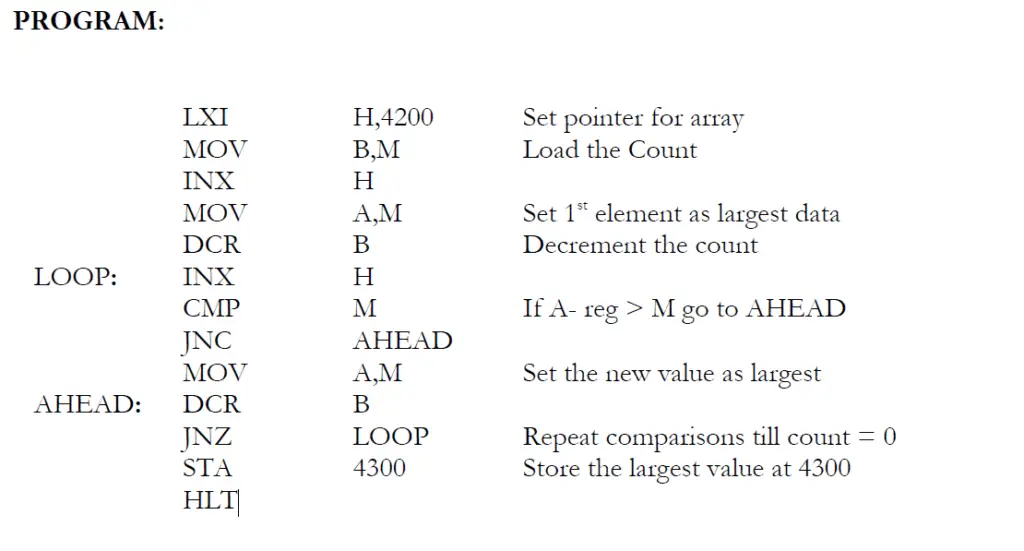# Find the largest number in an array of data using 8085 microprocessor

Last updated on March 9th, 2022 at 11:03 am

Let’s see how to find the largest number in an array of data using the 8085 instruction set.

## Algorithm to Find the largest number in an array of data using 8085 microprocessor

1) Load the address of the first element of the array in HL pair
2) Move the count to B – reg.
3) Increment the pointer
4) Get the first data in A – reg.
5) Decrement the count.
6) Increment the pointer

7) Compare the content of memory addressed by HL pair with that of A – reg.
8) If Carry = 0, go to step 10 or if Carry = 1 go to step 9
9) Move the content of memory addressed by HL to A – reg.
10) Decrement the count

11) Check for Zero of the count. If ZF = 0, go to step 6, or if ZF = 1 go to next step.
12) Store the largest data in memory.
13) Terminate the program.

## 8085 microprocessor program to Find the largest number in an array of data using 8085 microprocessor

LXI H,4200 //Set pointer for array
INX H
MOV A,M //Set 1st element as largest data
DCR B //Decrement the count
LOOP: INX H
CMP M //If A- reg > M go to AHEAD
MOV A,M //Set the new value as largest
JNZ LOOP // Repeat comparisons till count = 0
STA 4300 // Store the largest value at 4300
HLT8085 microprocessor program to Find the largest number in an array of data using 8085 microprocessor

## Observation

Input:

05 (4200) —– Array Size
0A (4201)
F1 (4202)
1F (4203)
26 (4204)
FE (4205)